# Chemical Equations

## Chemical Equations

You will need to know:

What a chemical reaction is.

The difference between word and symbol equations.

When chemical reactions take place, bonds between atoms break and atoms change places to form different chemicals. The two (or more) chemicals that react together are called reactants, the chemicals that are left at the end of the reaction are called products.Chemical equations can be used to describe what is happening in a chemical reaction.

They come in three wonderful forms:

Word Equations, such as: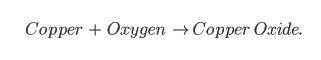Symbol Equations, such as: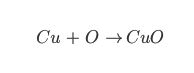Or even better: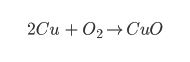Even though all three equations are showing the same chemical reaction, the last equation has the most information. We can see from the last equation that two particles of copper react with one particle of oxygen to form two atoms of copper oxide… like this!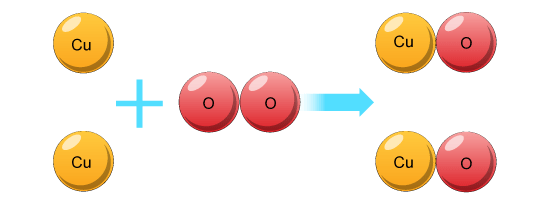## Balancing Equations

You will need to know:

What the big and small numbers mean in a symbol equation.

How to balance symbol equations.

You may have seen numbers being written in symbol equations, such as: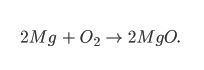This is a balanced symbol equation. Since atoms cannot be created or destroyed, there has to be the same amount of atoms at the start and end of the reaction.

Big Numbers:

The 2Mg means that there are two separate atoms of Magnesium reacting.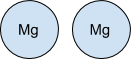Small Numbers:

The small 2 in the O2 means that there are two atoms of Oxygen bonded together to make one particle of O2.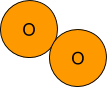#### Balancing Equations

There are times that you will need to balance equations. This is done by adding in big numbers to make sure that there are the same amount of particles at the start of the reaction and at the end.

Remember, you can add big numbers but you can NEVER add small numbers to an equation. Adding small numbers changes the type of atom, which makes it a different reaction all together!